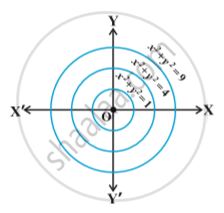Formation of a Differential Equation Whose General Solution is Given

notes

The equation is  x^2 + y^2 + 2x – 4y + 4 = 0 ... (1)
represents a circle having centre at (–1, 2) and radius 1 unit.
Differentiating equation (1) with respect to x, we get
(dy)/(dx) = (x + 1)/(2 - y) (y ≠ 2)    ...(2)
which is a differential equation. You will find later on  that this equation represents the family of circles and one member of the family is the circle given in equation (1).
Let us consider the equation:
x^2 + y^2 = r^2 ... (3)
By giving different values to r, we get different members of the family e.g. x^2 + y^2 = 1, x^2 + y^2 = 4, x^2 + y^2 = 9 etc   Fig .Thus, equation (3) represents a family of concentric circles centered at the origin and having different radii.
We are interested in finding a differential equation that is satisfied by each member of the family. The differential equation must be free from r because r is different for different members of the family. This equation is obtained by differentiating equation (3) with respect to x, i.e.,
2x + 2y (dy)/(dx) = 0 or  x + y (dy)/(dx) =0   ...(4)
which represents the family of concentric circles given by equation (3).

If you would like to contribute notes or other learning material, please submit them using the button below.

Video Tutorials

We have provided more than 1 series of video tutorials for some topics to help you get a better understanding of the topic.

Series 1

Series 2

Shaalaa.com

How to identify which form to apply Differential equations [00:30:25]
S
0%